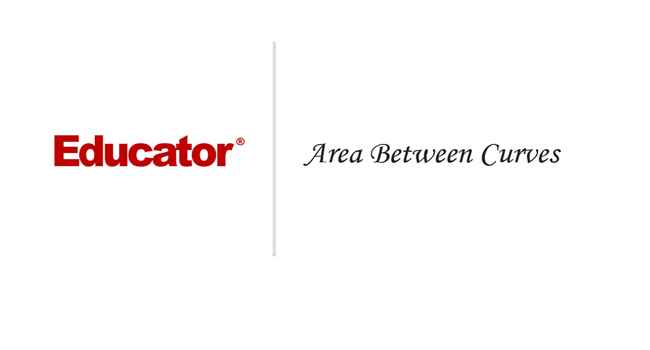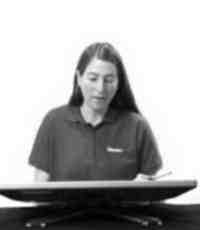Professor Switkes

Area Between Curves

Slide Duration:

Section 1: Overview of Functions
Review of Functions

26m 29s

Intro
0:00
What is a Function
0:10
Domain and Range
0:21
Vertical Line Test
0:31
Example: Vertical Line Test
0:47
Function Examples
1:57
Example: Squared
2:10
Example: Natural Log
2:41
Example: Exponential
3:21
Example: Not Function
3:54
Odd and Even Functions
4:39
Example: Even Function
5:10
Example: Odd Function
5:53
Odd and Even Examples
6:48
Odd Function
6:55
Even Function
8:43
Increasing and Decreasing Functions
10:15
Example: Increasing
10:42
Example: Decreasing
10:55
Increasing and Decreasing Examples
11:41
Example: Increasing
11:48
Example: Decreasing
12:33
Types of Functions
13:32
Polynomials
13:45
Powers
14:06
Trigonometric
14:34
Rational
14:50
Exponential
15:13
Logarithmic
15:29
Lecture Example 1
15:55
Lecture Example 2
17:51
-1
-2
Compositions of Functions

12m 29s

Intro
0:00
Compositions
0:09
Alternative Notation
0:32
Three Functions
0:47
Lecture Example 1
1:19
Lecture Example 2
3:25
Lecture Example 3
6:45
-1
-2
Section 2: Limits
Average and Instantaneous Rates of Change

20m 59s

Intro
0:00
Rates of Change
0:11
Average Rate of Change
0:21
Instantaneous Rate of Change
0:33
Slope of the Secant Line
0:46
Slope of the Tangent Line
1:00
Lecture Example 1
1:14
Lecture Example 2
6:36
Lecture Example 3
11:30
-1
-2
Limit Investigations

22m 37s

Intro
0:00
What is a Limit?
0:10
Lecture Example 1
0:56
Lecture Example 2
5:28
Lecture Example 3
9:27
-1
-2
Algebraic Evaluation of Limits

28m 19s

Intro
0:00
Evaluating Limits
0:09
Lecture Example 1
1:06
Lecture Example 2
5:16
Lecture Example 3
8:15
Lecture Example 4
12:58
-1
-2
Formal Definition of a Limit

23m 39s

Intro
0:00
Formal Definition
0:13
Template
0:55
Epsilon and Delta
1:24
Lecture Example 1
1:40
Lecture Example 2
9:20
-1
-2
Continuity and the Intermediate Value Theorem

19m 9s

Intro
0:00
Continuity
0:13
Continuous
0:16
Discontinuous
0:37
Intermediate Value Theorem
0:52
Example
1:22
Lecture Example 1
2:58
Lecture Example 2
9:02
-1
-2
Section 3: Derivatives, part 1
Limit Definition of the Derivative

22m 52s

Intro
0:00
Limit Definition of the Derivative
0:11
Three Versions
0:13
Lecture Example 1
1:02
Lecture Example 2
4:33
Lecture Example 3
6:49
Lecture Example 4
10:11
-1
-2
The Power Rule

26m 1s

Intro
0:00
Power Rule of Differentiation
0:14
Power Rule with Constant
0:41
Sum/Difference
1:15
Lecture Example 1
1:59
Lecture Example 2
6:48
Lecture Example 3
11:22
-1
-2
The Product Rule

14m 54s

Statement of the Product Rule
0:08
Lecture Example 1
0:41
Lecture Example 2
2:27
Lecture Example 3
5:03
-1
-2
The Quotient Rule

19m 17s

Intro
0:00
Statement of the Quotient Rule
0:07
Carrying out the Differentiation
0:23
Quotient Rule in Words
1:00
Lecture Example 1
1:19
Lecture Example 2
4:23
Lecture Example 3
8:00
-1
-2
Applications of Rates of Change

17m 43s

Intro
0:00
Rates of Change
0:11
Lecture Example 1
0:44
Lecture Example 2
5:16
Lecture Example 3
7:38
-1
-2
Trigonometric Derivatives

26m 58s

Intro
0:00
Six Basic Trigonometric Functions
0:11
Patterns
0:47
Lecture Example 1
1:18
Lecture Example 2
7:38
Lecture Example 3
12:15
Lecture Example 4
14:25
-1
-2
The Chain Rule

23m 47s

Intro
0:00
Statement of the Chain Rule
0:09
Chain Rule for Three Functions
0:27
Lecture Example 1
1:00
Lecture Example 2
4:34
Lecture Example 3
7:23
-1
-2
Inverse Trigonometric Functions

27m 5s

Intro
0:00
Six Basic Inverse Trigonometric Functions
0:10
Lecture Example 1
1:11
Lecture Example 2
8:53
Lecture Example 3
12:37
-1
-2
Equation of a Tangent Line

15m 52s

Intro
0:00
Point Slope Form
0:10
Lecture Example 1
0:47
Lecture Example 2
3:15
Lecture Example 3
6:10
-1
-2
Section 4: Derivatives, part 2
Implicit Differentiation

30m 5s

Intro
0:00
Purpose
0:09
Implicit Function
0:20
Lecture Example 1
0:32
Lecture Example 2
7:14
Lecture Example 3
11:22
Lecture Example 4
16:43
-1
-2
Higher Derivatives

13m 16s

Intro
0:00
Notation
0:08
First Type
0:19
Second Type
0:54
Lecture Example 1
1:41
Lecture Example 2
3:15
Lecture Example 3
4:57
-1
-2
Logarithmic and Exponential Function Derivatives

17m 42s

Intro
0:00
Essential Equations
0:12
Lecture Example 1
1:34
Lecture Example 2
2:48
Lecture Example 3
5:54
-1
-2
Hyperbolic Trigonometric Function Derivatives

14m 30s

Intro
0:00
Essential Equations
0:15
Six Basic Hyperbolic Trigc Functions
0:32
Six Basic Inverse Hyperbolic Trig Functions
1:21
Lecture Example 1
1:48
Lecture Example 2
3:45
Lecture Example 3
7:09
-1
-2
Related Rates

29m 5s

Intro
0:00
What Are Related Rates?
0:08
Lecture Example 1
0:35
Lecture Example 2
5:25
Lecture Example 3
11:54
-1
-2
Linear Approximation

23m 52s

Intro
0:00
Essential Equations
0:09
Linear Approximation (Tangent Line)
0:18
Example: Graph
1:18
Differential (df)
2:06
Delta F
5:10
Lecture Example 1
6:38
Lecture Example 2
11:53
Lecture Example 3
15:54
-1
-2
Section 5: Application of Derivatives
Absolute Minima and Maxima

18m 57s

Intro
0:00
Minimums and Maximums
0:09
Absolute Minima and Maxima (Extrema)
0:53
Critical Points
1:25
Lecture Example 1
2:58
Lecture Example 2
6:57
Lecture Example 3
10:02
-1
-2
Mean Value Theorem and Rolle's Theorem

20m

Intro
0:00
Theorems
0:09
Mean Value Theorem
0:13
Graphical Explanation
0:36
Rolle's Theorem
2:06
Graphical Explanation
2:28
Lecture Example 1
3:36
Lecture Example 2
6:33
Lecture Example 3
9:32
-1
-2
First Derivative Test, Second Derivative Test

27m 11s

Intro
0:00
Local Minimum and Local Maximum
0:14
Example
1:01
First and Second Derivative Test
1:26
First Derivative Test
1:36
Example
2:00
Second Derivative Test (Concavity)
2:58
Example: Concave Down
3:15
Example: Concave Up
3:54
Inconclusive
4:19
Lecture Example 1
5:23
Lecture Example 2
12:03
Lecture Example 3
15:54
-1
-2
L'Hopital's Rule

23m 9s

Intro
0:00
Using L'Hopital's Rule
0:09
Informal Definition
0:34
Lecture Example 1
1:27
Lecture Example 2
4:00
Lecture Example 3
5:40
Lecture Example 4
9:38
-1
-2
Curve Sketching

40m 16s

Intro
0:00
Collecting Information
0:15
Domain and Range
0:17
Intercepts
0:21
Symmetry Properties (Even/Odd/Periodic)
0:33
Asymptotes (Vertical/Horizontal/Slant)
0:45
Critical Points
1:15
Increasing/Decreasing Intervals
1:24
Inflection Points
1:38
Concave Up/Down
1:52
Maxima/Minima
2:03
Lecture Example 1
2:58
Lecture Example 2
10:52
Lecture Example 3
17:55
-1
-2
Applied Optimization

25m 37s

Intro
0:00
Real World Problems
0:08
Sketch
0:11
Interval
0:20
Rewrite in One Variable
0:26
Maximum or Minimum
0:34
Critical Points
0:42
Optimal Result
0:52
Lecture Example 1
1:05
Lecture Example 2
6:12
Lecture Example 3
13:31
-1
-2
Newton's Method

25m 13s

Intro
0:00
Approximating Using Newton's Method
0:10
Good Guesses for Convergence
0:32
Lecture Example 1
0:49
Lecture Example 2
4:21
Lecture Example 3
7:59
-1
-2
Section 6: Integrals
Approximating Areas and Distances

36m 50s

Intro
0:00
Three Approximations
0:12
Right Endpoint, Left Endpoint, Midpoint
0:22
Formulas
1:05
Velocity and Distance
1:35
Lecture Example 1
2:28
Lecture Example 2
12:10
Lecture Example 3
19:43
-1
-2
Riemann Sums, Definite Integrals, Fundamental Theorem of Calculus

22m 2s

Intro
0:00
Important Equations
0:22
Riemann Sum
0:28
Integral
1:58
Integrand
2:35
Limits of Integration (Upper Limit, Lower Limit)
2:43
Other Equations
3:05
Fundamental Theorem of Calculus
4:00
Lecture Example 1
5:04
Lecture Example 2
10:43
Lecture Example 3
13:52
-1
-2
Substitution Method for Integration

23m 19s

Intro
0:00
U-Substitution
0:13
Important Equations
0:30
Purpose
0:36
Lecture Example 1
1:30
Lecture Example 2
6:17
Lecture Example 3
9:00
Lecture Example 4
11:24
-1
-2
Section 7: Application of Integrals, part 1
Area Between Curves

19m 59s

Intro
0:00
Area Between Two Curves
0:12
Graphic Description
0:34
Lecture Example 1
1:44
Lecture Example 2
5:39
Lecture Example 3
8:45
-1
-2
Volume by Method of Disks and Washers

24m 22s

Intro
0:00
Important Equations
0:16
Equation 1: Rotation about x-axis (disks)
0:27
Equation 2: Two curves about x-axis (washers)
3:38
5:31
Lecture Example 1
6:05
Lecture Example 2
8:28
Lecture Example 3
11:55
-1
-2
Volume by Method of Cylindrical Shells

30m 29s

Intro
0:00
Important Equations
0:50
1:04
Equation 2: Rotation about y-axis (2 curves)
7:34
8:15
Lecture Example 1
8:57
Lecture Example 2
14:26
Lecture Example 3
18:15
-1
-2
Average Value of a Function

16m 31s

Intro
0:00
Important Equations
0:11
Origin of Formula
0:34
Lecture Example 1
2:51
Lecture Example 2
5:30
Lecture Example 3
8:13
-1
-2
Section 8: Extra
Graphs of f, f', f''

23m 58s

Intro
0:00
Slope Function of f(x)
0:41
Slope is Zero
0:53
Slope is Positive
1:03
Slope is Negative
1:13
Slope Function of f'(x)
1:31
Slope is Zero
1:42
Slope is Positive
1:48
Slope is Negative
1:54
Lecture Example 1
2:23
Lecture Example 2
8:06
Lecture Example 3
12:36
-1
-2
Slope Fields for Differential Equations

18m 32s

Intro
0:00
Things to Remember
0:13
Graphic Description
0:42
Lecture Example 1
1:44
Lecture Example 2
6:59
Lecture Example 3
9:46
-1
-2
Separable Differential Equations

17m 4s

Intro
0:00
Differential Equations
0:10
Focus on Exponential Growth/Decay
0:27
Separating Variables
0:47
Lecture Example 1
1:35
Lecture Example 2
6:41
Lecture Example 3
9:36
-1
-2
Bookmark & Share Embed

## Copy & Paste this embed code into your website’s HTML

Please ensure that your website editor is in text mode when you paste the code.
(In Wordpress, the mode button is on the top right corner.)
×
• - Allow users to view the embedded video in full-size.
Since this lesson is not free, only the preview will appear on your website.

• ## Related Books0 answersPost by Eti Sinha on September 8, 2014For additional example #5, is it possible to find the area underneath the curve by: Integral of ( 1/y ) dy, from 1/3 to 3? Using dy as opposed to dx?0 answersPost by Rufino Hernandez on July 5, 2014Asides from getting the area of this triangle using this method, you can also get it using another method. The Area of a triangle is square root of (s(s-a)(s-b)(s-c)). Where s=(1/2)(a+b+c). Where a is one side of the triangle, b is another side of the triangle, and c is the third side of the triangle. Since the Triangle's lower angle is the same as the angle made by the function y=|x|, it can be deduced that the angle made is 90 degrees. If it is a 90 degree triangle, then we can simply use the Area of a triangle to come to the conclusion of the area; A=(1/2)b*h, where b=base and h=height. Since we have the end points of the sides of the triangle, we can simply derive sides. For example, the right side has an endpoint of (2,2); y=2, x=2. Using this we can make a second triangle where the sides are 2 and 2 which can lead us to find the third side which is square root of (8) = 2 * square root of(2). We now know the length of the right side of the triangle. Using this same same method, we can derive the length of the base of the triangle (bottom left). The endpoint of this side is (-2/3, -2/3). So we can make a third triangle that has these sides and derive the length of the second side of the original triangle. This second side will be (-2/3)^2+(-2/3)^2=c^2 ==> (4/9)+(4/9)= c^2 ==> 8/9=c^2 ==> c= (2 * square root of (2))/3. Now since we have the height and base, we can simply put this into the Area of a triangle; A=(1/2)(base)(height) ==> A=(1/2)((2square root of (2))/2)(2 * square root of (2) ==> 4/3. Or we can solve for the third side of the triangle and input them into the (The Area of a triangle is square root of (s(s-a)(s-b)(s-c)). Where s=(1/2)(a+b+c). Where a is one side of the triangle, b is another side of the triangle, and c is the third side of the triangle.) equation and get the same results. This method will yield the same answer as the method stated in this video.0 answersPost by Fadel Hanoun on December 17, 2013What about when x = 5pi/4, where Tan = 1?0 answersPost by Troy Ling on February 4, 2011k= -15/161 answerLast reply by: Nopparat SAntisathapornWed Oct 6, 2010 2:36 AMPost by Nopparat SAntisathaporn on October 6, 2010Hi there, I don't know how to do number 7 in this test http://www.nzqa.govt.nz/nqfdocs/ncea-resource/exams/2007/90286-exm-07.pdf Can you please explain how to solve for K. Thank You.

### Area Between Curves

• I recommend always starting with a sketch and drawing in a “sample rectangle.”
• If an interval is not given, you may need to set the two functions equal in order to determine the interval involved.
• If two curves cross, then you will need to break up the integral into more than one integral.
• Sometimes, geometrical considerations can help you to check your results or even provide a non-calculus way to finish a problem.

### Area Between Curves

Find the area enclosed by y = 4 and y = x2
• First, we find the bounds. To do so, we set the equations equal to each other
• x2 = 4
• x = ±2
•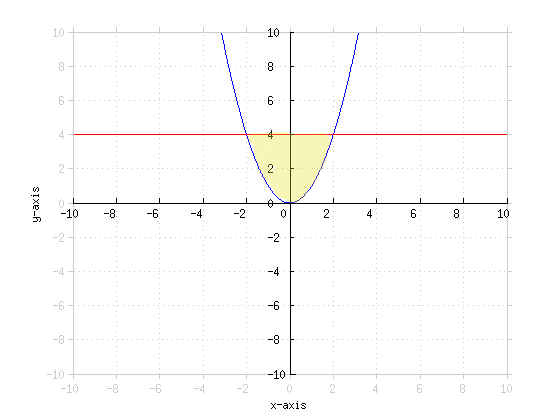• A = ∫−22 4 − x2  dx
• A = (4x − [(x3)/3]) |−22
• A = 8 − [8/3] − (−8 − [(−8)/3])
• A = 16 − [16/3]
A = [32/3]
Find the area under the velocity curve v(t) = 3t2 + t from t = 0 to t = 2
•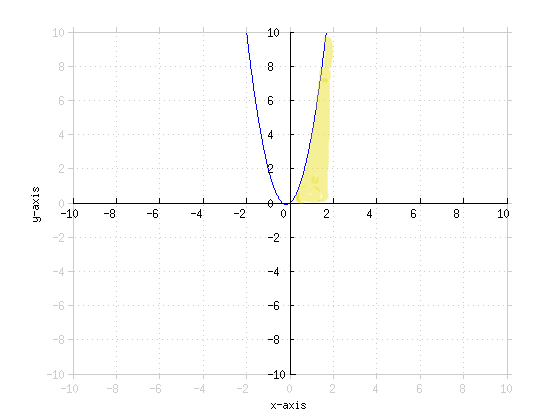• A = ∫02 3t2 + t − 0  dt
• The subtraction by zero has no effect. It is just to illustrate that we're taking the x-axis as the lower-bound.
• A = (t3 + [(t2)/2]) |02
• A = 8 + 2 − 0 − 0
• The area under a velocity curve also happens to be the displacement!
• t1t2 v(t) dt = x(t2) − x(t1)
A = 10
Find the area under y = ex from x = 0 to x = ln10
•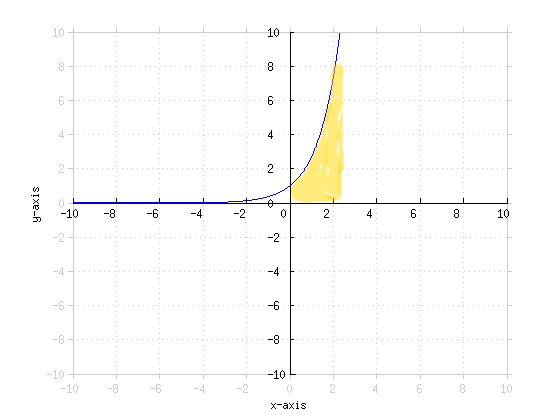• A = ∫0ln10 ex dx
• A = ex |0ln10
• A = eln10 − e0
• A = 10 − 1
• A = 9
A = 9
Find the area under y = e[t/3] from t = 0 to t = 6
•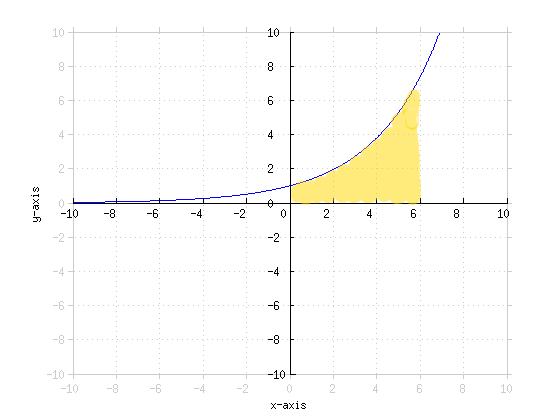• A = ∫06 e[t/3] dt
• u = [t/3]
• du = [1/3] dt
• A = 3 ∫06 eu du
• A = 3 e[t/3] |06
• A = 3 (e2 − e0)
A = 3(e2 − 1)
Find the area bounded by y = x2 and y = |x|
•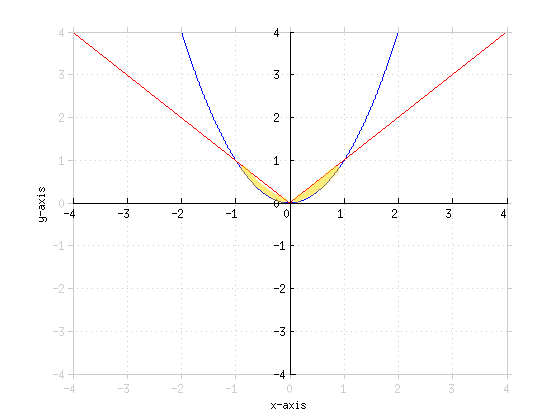• Find the bounds of the integral
• x2 = |x|
• x2 = √{x2}
• x4 = x2
• x2 = 1
• x = ±1
• y = |x| has different behavior on either side of zero. We can treat this as a piecewise function with the area as the sum of two different integrals
• A = ∫−10 −x − x2  dx + ∫01 x − x2  dx
• A = (−[(x2)/2] − [(x3)/3]) |−10 + ([(x2)/2] − [(x3)/3]) |01
• A = (−0 − 0) − (−[((−1)2)/2] − [((−1)3)/3]) + ([1/2] − [1/3]) − 0
• A = [1/2] − [1/3] + [1/2] − [1/3]
• A = 1 − [2/3]
A = [1/3]
Find the area bound by x = y3 and the y-axis from y = 0 to y = 2
•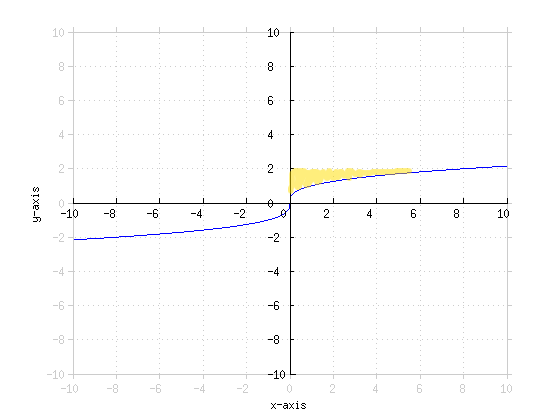• A = ∫02 y3 dy
• A = [(y4)/4] |02
• A = [(24)/4] − 0
A = 4
Find the area bound by y = sinx and the x-axis from x = 0 to x = 2π
•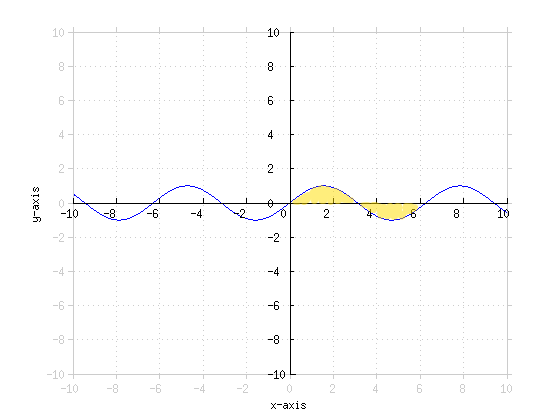• A = ∫0 sinx  dx
• A = − cosx |0
• A = −cos2π− (− cos0)
• A = −1 + 1 = 0
• An area of zero doesn't quite make sense here, so using the straight integral is insufficient. What we can do is treat this as two separate integrals, one where the area is above the x-axis and one where it is below and add their effective area.
• sinx = 0, x = 0, π, 2π in this interval.
• A = ∫0π sinx  dx + (−∫π sinx  dx)
• The negative sign is there to compensate for the "negative area"
• A = −cosx |0π + cosx |π
• A = − cosπ+ cos0 + cos2π− cosπ
• A = 1 + 1 + 1 + 1
A = 4
Find the area bound by x = y2 and y = x − 2
•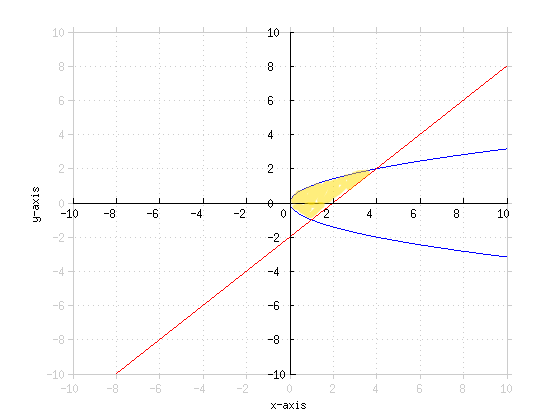• We could find the integral in terms of dx, but the we would have to split it up into at least two separate integrals. Besides, x = y2 is not a function of x (there are two outputs for every x input).
• x = y2
• x = y + 2
• Find the limits of the integral
• y2 = y + 2
• y2 − y − 2 = 0
• (y + 1)(y − 2) = 0
• y = 2, −1
• A = ∫−12 (y + 2) − y2 dy
• A = ([(y2)/2] + 2x − [(y3)/3]) |−12
• A = (2 + 4 − [8/3]) − ([1/2] − 2 + [1/3])
• A = 8 − [1/2] − [9/3]
• The area above the
A = [9/2]
Find the area bound by y = x2 + 1 and y = −x2 + 3
•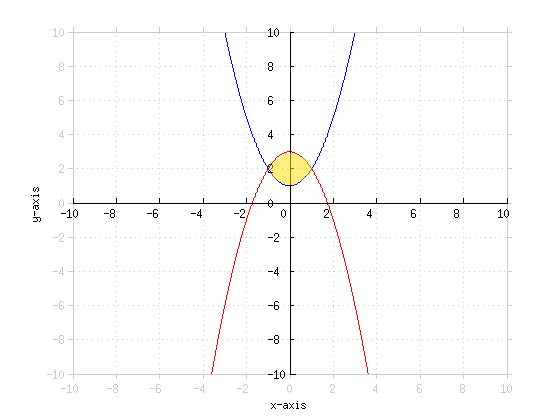• Find the limits of the integral
• x2 + 1 = −x2 + 3
• 2x2 = 2
• x2 = 1
• x = ±1
• A = ∫−11 (−x2 + 3) − (x2 + 1)  dx
• A = ∫−11 −2x2 + 2  dx
• A = (−[(2x3)/3] + 2x) |−11
• A = −[2/3] + 2 − (−[(−2)/3] − 2)
• A = −[2/3] + 2 − [2/3] + 2
• A = 4 − [4/3]
A = [8/3]
Find the area bound by y = x2 and y = 2x
•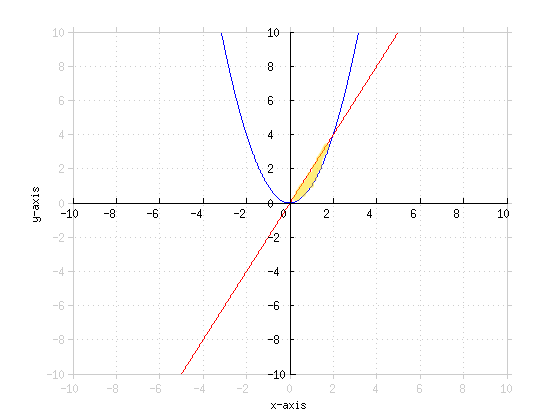• Find the limits
• x2 = 2x
• x2 − 2x = 0
• x(x − 2) = 0
• x = 0, 2
• A = ∫02 2x − x2 dx
• A = x2 − [(x3)/3] |02
• A = 4 − [8/3] − 0
A = [4/3]

*These practice questions are only helpful when you work on them offline on a piece of paper and then use the solution steps function to check your answer.

### Area Between Curves

Lecture Slides are screen-captured images of important points in the lecture. Students can download and print out these lecture slide images to do practice problems as well as take notes while watching the lecture.

• Intro 0:00
• Area Between Two Curves 0:12
• Graphic Description
• Lecture Example 1 1:44
• Lecture Example 2 5:39
• Lecture Example 3 8:45

OR

### Start Learning Now

Our free lessons will get you started (Adobe Flash® required).